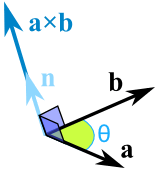Definition of

# Cross ProductA way of multiplying two vectors:

a × b = |a| |b| sin(θ) n

Where || means "the magnitude (length) of"
θ is the angle between the vectors
and n is the unit vector at right angles to both a and b

Example: the lengths of two vectors are 3 and 4, and the angle between them is 30°:
a × b = 3 × 4 × sin(30°) × n = 6n